﻿

### Some sporadic translation planes of order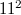$11^2$

#### Abstract

In \cite{PK}, the authors constructed a translation plane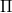$\Pi$ of order$11^2$ arising from replacement of a sporadic chain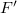$F'$ of reguli in a regular spread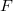$F$ of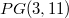$PG(3,11)$. They also showed that two more non isomorphic translation planes, called$\Pi_1$ and$\Pi_{13}$, arise respectively by derivation and double derivation in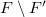$F\setminus F'$ which correspond to a further replacement of a regulus with its opposite regulus and a pair of reguli with their opposite reguli, respectively.  In \cite{AL}, the authors proved that the translation complement of$\Pi$ contains a subgroup isomorphic to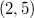$\SL(2,5)$. Here, the full collineation group of each of the planes$\Pi$,$\Pi_1$ and$\Pi_{13}$ is determined.

DOI Code: 10.1285/i15900932v29n1supplp121

Keywords:
Translation plane; Replacement; Collineation; Chain of circles

Full Text: PDF